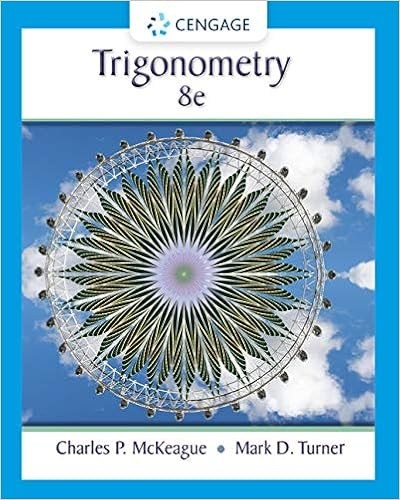# X 5 y 116 c x 5 y 32 dx 5 y 64 4 classify the

• Homework Help
• 20

This preview shows page 13 - 18 out of 20 pages.

##### We have textbook solutions for you!
The document you are viewing contains questions related to this textbook.The document you are viewing contains questions related to this textbook.
Chapter 8 / Exercise 102
Trigonometry
McKeague/TurnerExpert Verified
x = 5, y = 116°c.x = 5, y = 32°d.x = 5, y = 64°4.Classify the triangle by sides and angles.a.scalene, obtuseb.isosceles, acutec.scalene, acuteGeometry-Congruent Triangles~13~NJCTL.orgy X 532 3281210
##### We have textbook solutions for you!
The document you are viewing contains questions related to this textbook.The document you are viewing contains questions related to this textbook.
Chapter 8 / Exercise 102
Trigonometry
McKeague/TurnerExpert Verified
RPQS
U
5.If ∆ D EF∆ PQ R, one set of corresponding sides are:
6.Are the triangles congruent – if so, by which congruence postulate/theorem?
7.By which postulate/theorem, if any, are the two triangles congruent?a.ASAc. SASb.AASd. Not congruent
8.State the third congruence needed to make ∆ A BC∆ D EFtrue by SAS congruence.
E
¿
¿
JLGAIHB
AMXINz40xlist the congruence postulate or theorem, if anylist the corresponding parts write a congruence statement, if any11.12.13.Solve for z. ABCDX9.What information must be true for ASA congruencebetween the two triangles?
¿
10.State the third congruence needed to make ∆ X Y Z∆ PQ Rtrue by ASA congruence.
X
Q
¿
Short Constructed Response– Write the correct answer for each question. No partial credit will be given.#11- 12 For the triangles in the diagram:list the congruence postulate or theorem, if anylist the corresponding parts write a congruence statement, if any11.12.13.Solve for z. ABCDXGeometry-Congruent Triangles~15~NJCTL.orgKJLGAIHB
AMXINExtended Constructed Response- Solve the problem, showing all work. Partial credit may be given.14.Write a two-column or flow proof.Geometry-Congruent Triangles~16~NJCTL.org2x-10
Answers1.Scalene2.Isosceles3.Isosceles4.Equilateral 5.Scalene6.Right7.Equiangular & acute8.Obtuse9.Acute10.Obtuse11.Never12.Never13.Sometimes14.Sometimes15.Sometimes16.Sides: Isosceles, Angles: Acute17.Sides: Scalene, Angles: Obtuse18.Sides: Isosceles, Angles: Obtuse19.Sides: Scalene, Angles: Right20.Sides: Isosceles, Angles: Obtuse21.Scalene22.Isosceles23.Equilateral 24.Isosceles25.Scalene26.Equiangular & acute27.Right28.Obtuse29.Obtuse30.Acute31.Never32.Never33.Sometimes34.Never35.Sometimes36.Sides: Scalene, Angles: Right37.Sides: Scalene, Angles: Obtuse38.Sides: Isosceles, Angles: Obtuse39.Sides: Isosceles, Angles: Acute40.Sides: Isosceles, Angles: Acute41.X=58°42.X=33°43.X=23°44.X=30°45.X=79°, z=144°, y=55°46.X=1247.X=2748.(PROBLEM MISSING)
•••